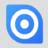How to prevent calculated field to been updated?

I have a table with some data and each time I add a new line with new values, the last entry become ''actual''. So I can have a follow like : taxes were 5% but since last week it s 6%....

The problem is that I have another table with calculated fields and those fields takes the actual data to be sure to have the good data... but I wish the existing entry to not recalculate if I update taxes value...the past entry must stay to 5% and the new one 6%...

How can I do?

thanks!Hi,

Calculated field will calculate always the current result of the inserted formula.

If you want to save the calculated results you need to calculate the value with a formula in a trigger or in a button and write it in a normal field (e. g. number or text).

Kind regards, Jörg